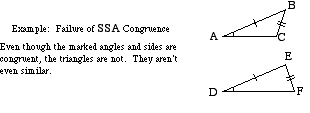index: click on a letter A B C D E F G H I J K L M N O P Q R S T U V W X Y Z A to Z index index: subject areas numbers & symbols sets, logic, proofs geometry algebra trigonometry advanced algebra & pre-calculus calculus advanced topics probability & statistics real world applications multimedia entrieswww.mathwords.com about mathwords website feedback

 SSA Side-side-angle. This is NOT one of the congruence tests or similarity tests for triangles. The fact that two triangles have congruent corresponding angles and sides as shown below does not mean that the triangles are congruent. Note: When a SSA correspondence exists there are often two possible configurations for the triangles.See also Similar